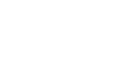Railo 4.2 Reference
Choose a function:

## Function EXP

Calculates the exponent whose base is e that represents number.
The constant e equals 2.71828182845904, the base of the natural
logarithm. This function is the inverse of Log, the natural
logarithm of number.

## Example

`exp(number number):number`

## Arguments

The arguments for this function are set. You can not use other arguments except the following ones.
Name Type Required Description
number number  Yes Exponent to apply to the base e